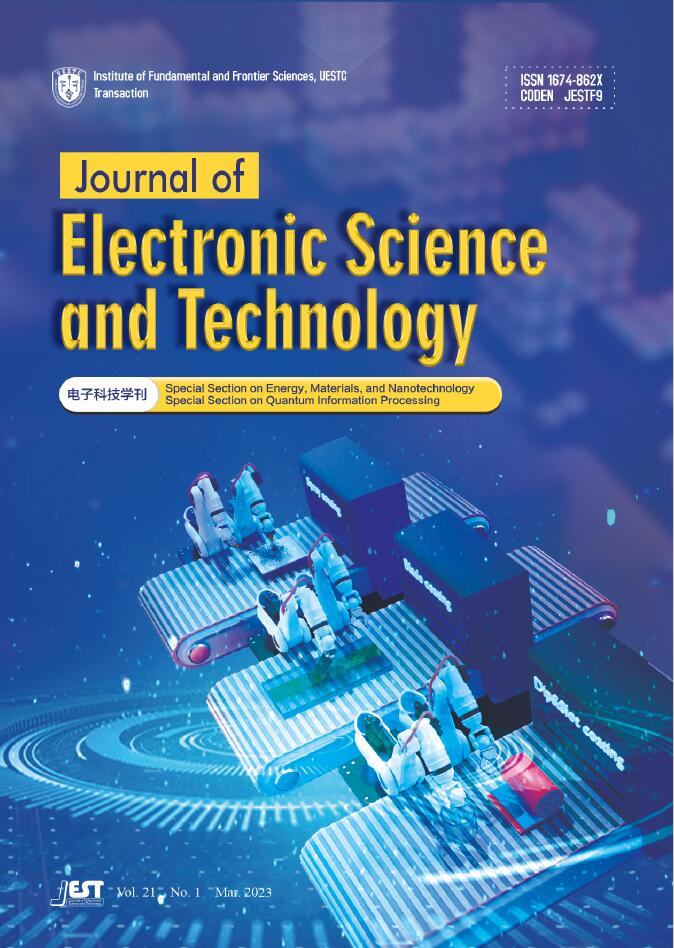Display Method:
Column
Display Method:
2023, 21(1).
[Abstract](13) [PDF 12708KB](5)
Abstract:
2023, 21(1): 1-1.
[Abstract](13) [FullText HTML](10) [PDF 133KB](4)
Abstract:
2023, 21(1): 100189.   doi: 10.1016/j.jnlest.2023.100189
[Abstract](14) [FullText HTML](9) [PDF 19588KB](3)
Abstract:
Colloidal quantum dots (CQDs) are of great interest to photovoltaic (PV) technologies as they possess the benefits of solution-processability, size-tunability, and roll-to-roll manufacturability, as well as unique capabilities to harvest near-infrared (NIR) radiation. During the last decade, lab-scale CQD solar cells have achieved rapid improvement in the power conversion efficiency (PCE) from ~1% to 18%, which will potentially exceed 20% in the next few years and approach the performance of other PV technologies, such as perovskite solar cells and organic solar cells. In the meanwhile, CQD solar cells exhibit long lifetimes either under shelf storage or continuous operation, making them highly attractive to industry. However, in order to meet the industrial requirements, mass production techniques are necessary to scale up the fabrication of those lab devices into large-area PV modules, such as roll-to-toll coating. This paper reviews the recent developments of large-area CQD solar cells with a focus on various fabrication methods and their principles. It covers the progress of typical large-area coating techniques, including spray coating, blade coating, dip coating, and slot-die coating. It also discusses next steps and new strategies to accomplish the ultimate goal of the low-cost large-area fabrication of CQD solar cells and emphasizes how artificial intelligence or machine learning could facilitate the developments of CQD solar cell research.
2023, 21(1): 100183.   doi: 10.1016/j.jnlest.2023.100183
Abstract:
Quantum-dot cellular automata (QCA) is an emerging computational paradigm which can overcome scaling limitations of the existing complementary metal oxide semiconductor (CMOS) technology. The existence of defects cannot be ignored, considering the fabrication of QCA devices at the molecular level where it could alter the functionality. Therefore, defects in QCA devices need to be analyzed. So far, the simulation-based displacement defect analysis has been presented in the literature, which results in an increased demand in the corresponding mathematical model. In this paper, the displacement defect analysis of the QCA main primitive, majority voter (MV), is presented and carried out both in simulation and mathematics, where the kink energy based mathematical model is applied. The results demonstrate that this model is valid for the displacement defect in QCA MV.
2023, 21(1): 100184.   doi: 10.1016/j.jnlest.2023.100184
[Abstract](17) [FullText HTML](11) [PDF 17553KB](2)
Abstract:
For the anti-interference/denoise purpose, it usually requires minimizing the sidelobe level (SLL) of a wide-beam pattern with a desired low nulling level (NL) in the nulling region. To realize such an objective, the shaped-beam pattern synthesis (SBPS) is the most commonly used approach. However, since the SBPS problem focuses on synthesizing a predetermined beam shape, the minimum SLL via this approach cannot ensure to obtain the maximum power gain. Conversely, it cannot obtain the lowest SLL with a certain power gain requirement. Based on such consideration, this paper tries to further minimize SLL of a wide-beam pattern with a desired low NL nulling region, by solving the power gain pattern synthesis (PGPS) problem. The PGPS problem selects the array excitation by directly optimizing the power gain. Hence, it has the potential to reduce SLL, when achieving the equal mainlobe power gain constraint via SBPS. An iterative algorithm which converts the primal optimization problem into convex sub-problems is proposed, resulting in an effective problem-solving scheme. Numerical simulations demonstrate the proposed algorithm can obtain about 10-dB lower SLL than the existing algorithms.
2023, 21(1): 100192.   doi: 10.1016/j.jnlest.2023.100192
[Abstract](34) [FullText HTML](21) [PDF 18327KB](2)
Abstract:
This paper proposes low-cost yet high-accuracy direction of arrival (DOA) estimation for the automotive frequency-modulated continuous-wave (FMCW) radar. The existing subspace-based DOA estimation algorithms suffer from either high computational costs or low accuracy. We aim to solve such contradictory relation between complexity and accuracy by using randomized matrix approximation. Specifically, we apply an easily-interpretable randomized low-rank approximation to the covariance matrix (CM) and approximately compute its subspaces. That is, we first approximate CM \begin{document}${\bf{R} }\in {\mathbb{C}}^{M\times M}$\end{document} through three sketch matrices, in the form of \begin{document}$\mathbf{R}\approx \mathbf{Q}\mathbf{B}{\mathbf{Q}}^{\mathrm{H}}$\end{document}. Here the matrix \begin{document}$\mathbf{Q}\in {\mathbb{C}}^{M\times z}$\end{document} contains the orthonormal basis for the range of the sketch matrix \begin{document}$\mathbf{C}\in {\mathbb{C}}^{M\times z}$\end{document} which is extracted from \begin{document}$\mathbf{R}$\end{document} using randomized uniform column sampling and \begin{document}$\mathbf{B}\in {\mathbb{C}}^{z\times z}$\end{document} is a weight-matrix reducing the approximation error. Relying on such approximation, we are able to accelerate the subspace computation by the orders of the magnitude without compromising estimation accuracy. Furthermore, we drive a theoretical error bound for the suggested scheme to ensure the accuracy of the approximation. As validated by the simulation results, the DOA estimation accuracy of the proposed algorithm, efficient multiple signal classification (E-MUSIC), is high, closely tracks standard MUSIC, and outperforms the well-known algorithms with tremendously reduced time complexity. Thus, the devised method can realize high-resolution real-time target detection in the emerging multiple input and multiple output (MIMO) automotive radar systems.
2023, 21(1): 100191.   doi: 10.1016/j.jnlest.2023.100191
[Abstract](23) [FullText HTML](14) [PDF 18169KB](3)
Abstract:
A compressive near-field millimeter wave (MMW) imaging algorithm is proposed. From the compressed sensing (CS) theory, the compressive near-field MMW imaging process can be considered to reconstruct an image from the under-sampled sparse data. The Gini index (GI) has been founded that it is the only sparsity measure that has all sparsity attributes that are called Robin Hood, Scaling, Rising Tide, Cloning, Bill Gates, and Babies. By combining the total variation (TV) operator, the GI-TV mixed regularization introduced compressive near-field MMW imaging model is proposed. In addition, the corresponding algorithm based on a primal-dual framework is also proposed. Experimental results demonstrate that the proposed GI-TV mixed regularization algorithm has superior convergence and stability performance compared with the widely used l1-TV mixed regularization algorithm.
2023, 21(1): GFA-1-GFA-3.
[Abstract](16) [FullText HTML](11) [PDF 253KB](3)
Abstract:
2023, 21(1): CFP-AT.
[Abstract](14) [FullText HTML](9) [PDF 3519KB](2)
Abstract:
2023, 21(1): CFP-Bi.
[Abstract](11) [FullText HTML](7) [PDF 214KB](2)
Abstract:Current Issue

Mar. 2023 Vol. 21 No. 1